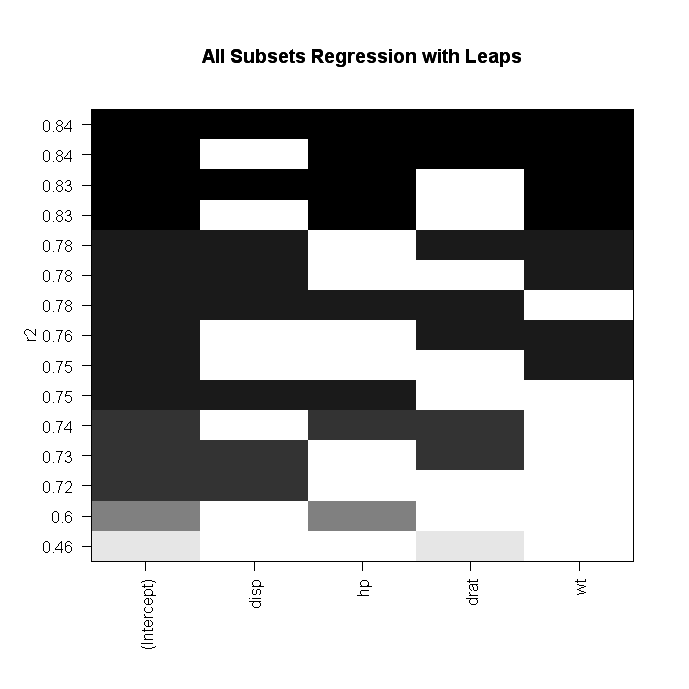# Subset Selection Regression

There are many different ways in which the variables of a regression model can be selected. In this post, we look at several common ways in which to selectvariables or features for a regression model. In particular, we look at the following.

• Best subset regression
• Stepwise selection

Best Subset Regression

Best subset regression fits a regression model for every possible combination of variables. The “best” model can be selected based on such criteria as the adjusted r-square, BIC (Bayesian Information Criteria), etc.

The primary drawback to best subset regression is that it becomes impossible to compute the results when you have a large number of variables. Generally, when the number of variables exceeds 40 best subset regression becomes too difficult to calculate.

Stepwise Selection

Stepwise selection involves adding or taking away one variable at a time from a regression model. There are two forms of stepwise selection and they are forward and backward selection.

In forward selection, the computer starts with a null model ( a model that calculates the mean) and adds one variable at a time to the model. The variable chosen is the one the provides the best improvement to the model fit. This process reduces greatly the number of models that need to be fitted in comparison to best subset regression.

Backward selection starts the full model and removes one variable at a time based on which variable improves the model fit the most. The main problem with either forward or backward selection is that the best model may not always be selected in this process. In addition, backward selection must have a sample size that is larger than the number of variables.

Deciding Which to Choose

Best subset regression is perhaps most appropriate when you have a small number of variables to develop a model with, such as less than 40. When the number of variables grows forward or backward selection are appropriate. If the sample size is small forward selection may be a better choice. However, if the sample size is large as in the number of examples is greater than the number of variables it is now possible to use backward selection.

Conclusion

The examples here are some of the most basic ways to develop a regression model. However, these are not the only ways in which this can be done. What these examples provide is an introduction to regression model development. In addition, these models provide some sort of criteria for the addition or removal of a variable based on statistics rather than intuition.